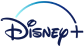## FANDOM

239,736 Pages

```local monthAliases = {
["1"] = "January",
["early january"] = "January",
["mid january"] = "January",
["late january"] = "January",
["january"] = "January",
["jan"] = "January",
["1x"] = "January",
["early januaryx"] = "January",
["mid januaryx"] = "January",
["late januaryx"] = "January",
["januaryx"] = "January",
["janx"] = "January",
["2"] = "February",
["early february"] = "February",
["mid february"] = "February",
["late february"] = "February",
["february"] = "February",
["feb"] = "February",
["2x"] = "February",
["early februaryx"] = "February",
["mid februaryx"] = "February",
["late februaryx"] = "February",
["februaryx"] = "February",
["febx"] = "February",
["3"] = "March",
["early march"] = "March",
["mid march"] = "March",
["late march"] = "March",
["march"] = "March",
["mar"] = "March",
["3x"] = "March",
["early marchx"] = "March",
["mid marchx"] = "March",
["late marchx"] = "March",
["marchx"] = "March",
["marx"] = "March",
["4"] = "April",
["early april"] = "April",
["mid april"] = "April",
["late april"] = "April",
["april"] = "April",
["apr"] = "April",
["4x"] = "April",
["early aprilx"] = "April",
["mid aprilx"] = "April",
["late aprilx"] = "April",
["aprilx"] = "April",
["aprx"] = "April",
["5"] = "May",
["early may"] = "May",
["mid may"] = "May",
["late may"] = "May",
["may"] = "May",
["5x"] = "May",
["early mayx"] = "May",
["mid mayx"] = "May",
["late mayx"] = "May",
["mayx"] = "May",
["6"] = "June",
["early june"] = "June",
["mid june"] = "June",
["late june"] = "June",
["june"] = "June",
["jun"] = "June",
["6x"] = "June",
["early junex"] = "June",
["mid junex"] = "June",
["late junex"] = "June",
["junex"] = "June",
["junx"] = "June",
["7"] = "July",
["early july"] = "July",
["mid july"] = "July",
["late july"] = "July",
["july"] = "July",
["jul"] = "July",
["7x"] = "July",
["early julyx"] = "July",
["mid julyx"] = "July",
["late julyx"] = "July",
["julyx"] = "July",
["julx"] = "July",
["8"] = "August",
["early august"] = "August",
["mid august"] = "August",
["late august"] = "August",
["august"] = "August",
["aug"] = "August",
["8x"] = "August",
["early augustx"] = "August",
["mid augustx"] = "August",
["late augustx"] = "August",
["augustx"] = "August",
["augx"] = "August",
["9"] = "September",
["early september"] = "September",
["mid september"] = "September",
["late september"] = "September",
["september"] = "September",
["sept"] = "September",
["sep"] = "September",
["9x"] = "September",
["early septemberx"] = "September",
["mid septemberx"] = "September",
["late septemberx"] = "September",
["septemberx"] = "September",
["septx"] = "September",
["sepx"] = "September",
["10"] = "October",
["early october"] = "October",
["mid october"] = "October",
["late october"] = "October",
["october"] = "October",
["oct"] = "October",
["10x"] = "October",
["early octoberx"] = "October",
["mid octoberx"] = "October",
["late octoberx"] = "October",
["octoberx"] = "October",
["octx"] = "October",
["11"] = "November",
["early november"] = "November",
["mid november"] = "November",
["late november"] = "November",
["november"] = "November",
["nov"] = "November",
["11x"] = "November",
["early novemberx"] = "November",
["mid novemberx"] = "November",
["late novemberx"] = "November",
["novemberx"] = "November",
["novx"] = "November",
["12"] = "December",
["early december"] = "December",
["mid december"] = "December",
["late december"] = "December",
["december"] = "December",
["dec"] = "December",
["12x"] = "December",
["early decemberx"] = "December",
["mid decemberx"] = "December",
["late decemberx"] = "December",
["decemberx"] = "December",
["decx"] = "December",
["spring"] = "March",
["springx"] = "Spring",
["summer"] = "June",
["summerx"] = "Summer",
["autumn"] = "September",
["fall"] = "September",
["autumnx"] = "Fall",
["fallx"] = "Fall",
["winter"] = "December",
["winterx"] = "Winter"
}

local monthNumbers = {
['1'] = '01',
['early january'] = '01',
['mid january'] = '01',
['late january'] = '01',
['january'] = '01',
['jan'] = '01',
['2'] = '02',
['early february'] = '02',
['mid february'] = '02',
['late february'] = '02',
['february'] = '02',
['feb'] = '02',
['3'] = '03',
['early march'] = '03',
['mid march'] = '03',
['late march'] = '03',
['march'] = '03',
['mar'] = '03',
['4'] = '04',
['early april'] = '04',
['mid april'] = '04',
['late april'] = '04',
['april'] = '04',
['apr'] = '04',
['5'] = '05',
['early may'] = '05',
['mid may'] = '05',
['late may'] = '05',
['may '] = '05',
['may'] = '05',
['6'] = '06',
['early june'] = '06',
['mid june'] = '06',
['late june'] = '06',
['june'] = '06',
['jun'] = '06',
['7'] = '07',
['early july'] = '07',
['mid july'] = '07',
['late july'] = '07',
['july'] = '07',
['jul'] = '07',
['8'] = '08',
['early august'] = '08',
['mid august'] = '08',
['late august'] = '08',
['august'] = '08',
['aug'] = '08',
['9'] = '09',
['early september'] = '09',
['mid september'] = '09',
['late september'] = '09',
['september'] = '09',
['sept'] = '09',
['sep'] = '09',
['10'] = '10',
['early october'] = '10',
['mid october'] = '10',
['late october'] = '10',
['october'] = '10',
['oct'] = '10',
['11'] = '11',
['early november'] = '11',
['mid november'] = '11',
['late november'] = '11',
['november'] = '11',
['nov'] = '11',
['12'] = '12',
['early december'] = '12',
['mid december'] = '12',
['late december'] = '12',
['december'] = '12',
['dec'] = '12',
['spring'] = '03',
['summer'] = '06',
['fall'] = '09',
['winter'] = '12'
}

return { monthAliases, monthNumbers }```
Community content is available under CC-BY-SA unless otherwise noted.